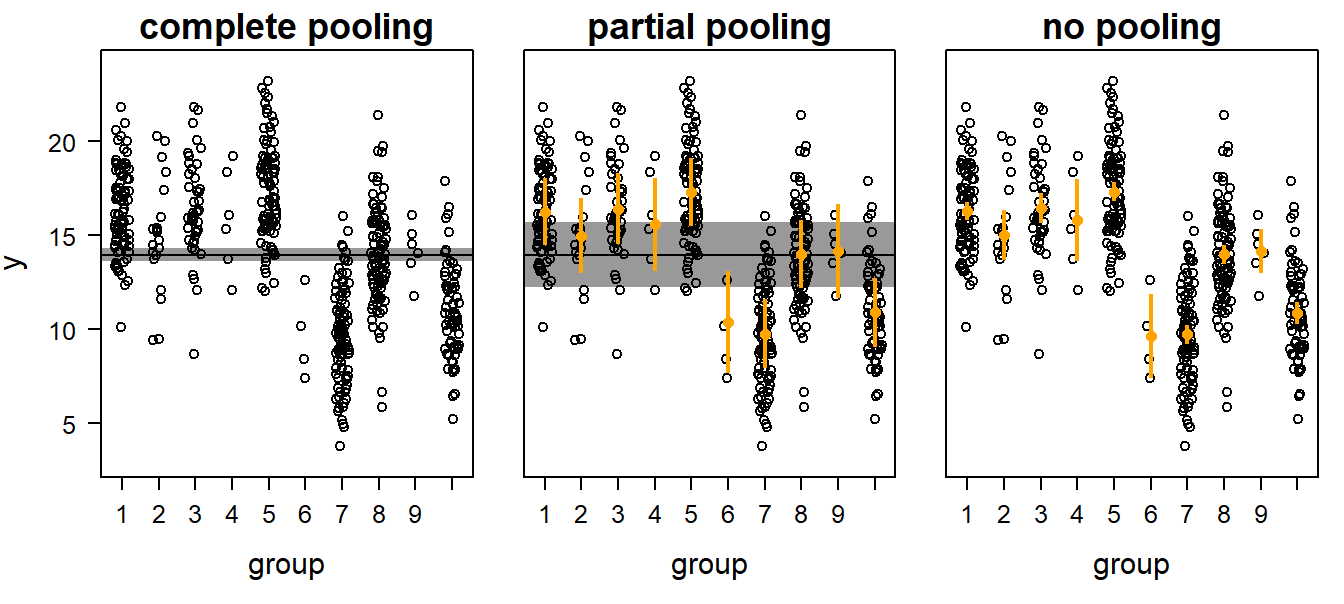# 13 Linear mixed effect models

## 13.1 Background

### 13.1.1 Why Mixed Effects Models?

Mixed effects models (or hierarchical models; see Gelman & Hill, 2007, for a discussion on the terminology) are used to analyze nonindependent, grouped, or hierarchical data. For example, when we measure growth rates of nestlings in different nests by taking mass measurements of each nestling several times during the nestling phase, the measurements are grouped within nestlings (because there are repeated measurements of each) and the nestlings are grouped within nests. Measurements from the same individual are likely to be more similar than measurements from different individuals, and individuals from the same nest are likely to be more similar than nestlings from different nests. Measurements of the same group (here, the “groups” are individuals or nests) are not independent. If the grouping structure of the data is ignored in the model, the residuals do not fulfill the independence assumption. Predictor variables can be measured on different hierarchical levels. For example, in each nest some nestlings were treated with a hormone implant whereas others received a placebo. Thus, the treatment is measured at the level of the individual, while clutch size is measured at the level of the nest. Clutch size was measured only once per nest but entered in the data file more than once (namely for each individual from the same nest). Similarly, all observations of one individual have the same value for treatment (but different values for individual measures such as weight). This results in pseudoreplication if we do not account for the hierarchical data structure in the model. Mixed models allow modeling of the hierarchical structure of the data and, therefore, account for pseudoreplication. Mixed models are further used to analyze variance components. For example, when the nestlings were cross-fostered so that they were not raised by their genetic parents, we would like to estimate the proportions of the variance (in a measurement, e.g., wing length) that can be assigned to genetic versus to environmental differences. Mixed models contain fixed and random effects. Note that, by definition, fixed and random effects are factors. Fixed effects have a finite (“fixed”) number of levels; for example, the factor “sex” has the levels male and female and (in many studies) nothing more. In contrast, random effects have a theoretically infinite number of levels of which we have measured a random sample. For example, we have measured 10 nests, but there are many more nests in the world that we have not measured. Normally, fixed effects have a low number of levels whereas random effects have a large number of levels (at least 3!). For fixed effects we are interested in the specific differences between levels (e.g., between males and females), whereas for random effects we are only interested in the between-level (¼ between-group, e.g., between-nest) variance rather than in differences between specific levels (e.g., nest A versus nest B). Typical fixed effects are: treatment, sex, age classes, or season. Typical random effects are: nest, individual, field, school, or study plot. It depends sometimes on the aim of the study whether a factor should be treated as fixed or random. When we would like to compare the average size of a corn cob between specific regions, then we include region as a fixed factor. However, when we would like to measure the size of a corn cob for a larger area within which we have measurements from a random sample of regions, then we treat region as a random factor.

### 13.1.2 Random Factors and Partial Pooling

In a model with fixed factors, the differences of the group means to the mean of the reference group are separately estimated as model parameters. This produces k  1 (independent) model parameters, where k ¼ number of groups (or number of factor levels). In contrast, for a random factor, only one parameter, namely the between-group variance, is estimated. To estimate this variance, we look at the differences of the group means to the population mean; that is, we look at k differences from the population mean. These k differences are not independent. They are assumed to be realizations of the same (in most cases normal) distribution with mean zero. They are like residuals, and we usually call them bg; each is the difference, b, between the mean of group, g, and the mean of all groups. The variance of the bg values is the between-group variance. Treating a factor as a random factor is equivalent to partial pooling of the data. There are three different ways to obtain means for grouped data. First, the grouping structure of the data can be ignored. This is called complete pooling (left panel in Figure (pooling)).Figure 13.1: Three possibilities to obtain group means for grouped data byi: complete pooling,partial pooling, and no pooling. Open symbols = data, orange dots with vertical bars = group means with 95% credible intervals, horizontal black line with shaded interval = population mean with 95% credible interval.

Second, group means may be estimated separately for each group. In this case, the data from all other groups are ignored when estimating a group mean. No pooling occurs in this case (right panel in Figure 7-1). Third, the data of the different groups can be partially pooled (i.e., treated as a random effect). Thereby, the group means are weighted averages of the population mean and the unpooled group means. The weights are proportional to sample size and the inverse of the variance (see Gelman & Hill, 2007, p. 252).